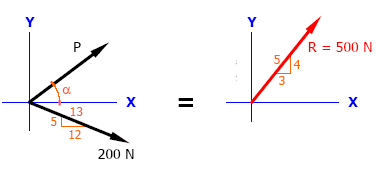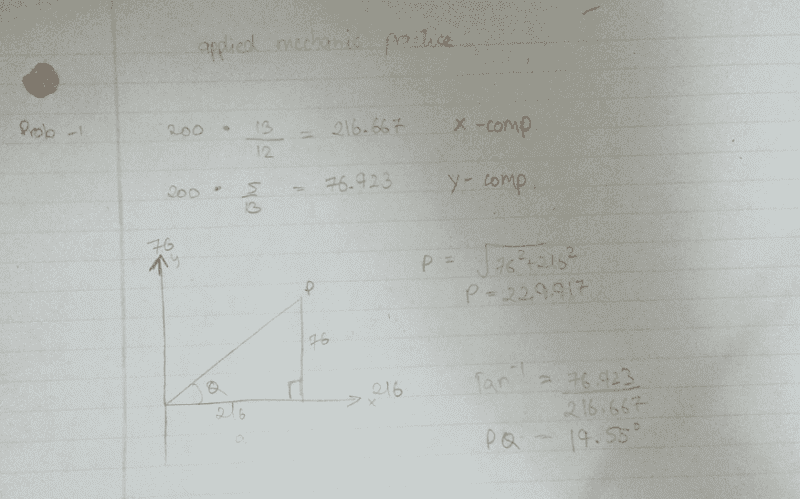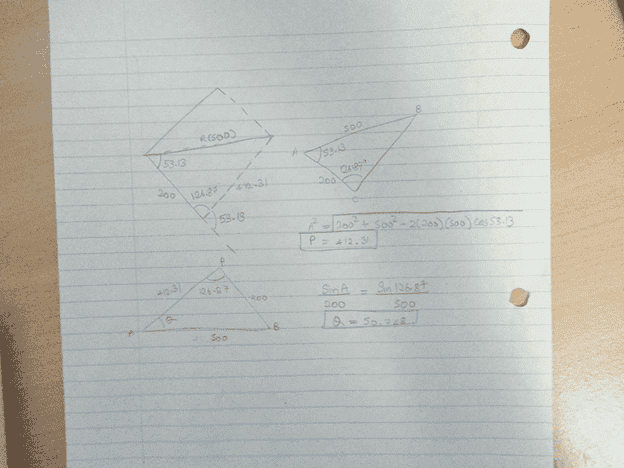# Moments. Finding angle and force

## Homework Statement

Two Forces are acting concurrently and their resultant is 500 N as shown in figure on the right. Determine P and the angle P makes with the x-axis?2. The attempt at a solutionI'm afraid it isn't. For one, you're x-component is larger in magnitude that the original force. And you haven't made use of the resultant of 500 N.
Use an equation such that the resultant of 'P' and the 200N vector is the 500N vector.

I'm afraid it isn't. For one, you're x-component is larger in magnitude that the original force. And you haven't made use of the resultant of 500 N.
Use an equation such that the resultant of 'P' and the 200N vector is the 500N vector.

I think this is what you mean.